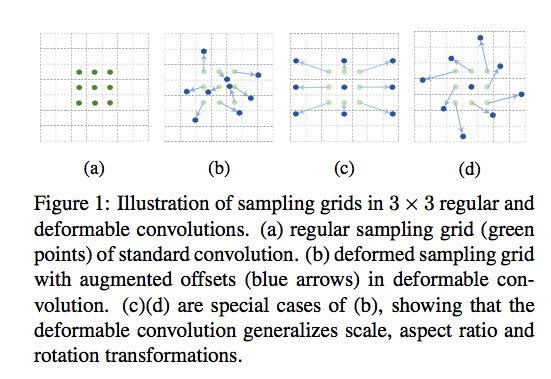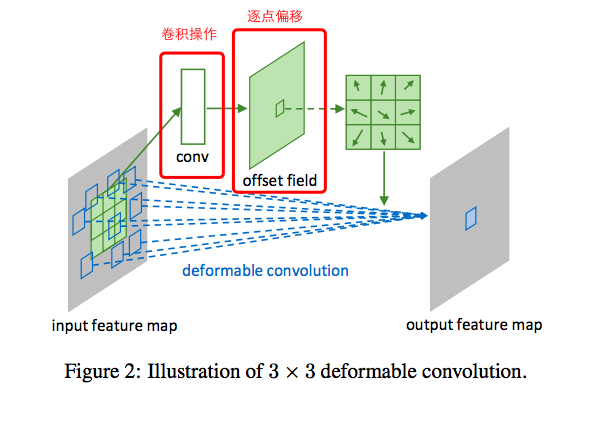Deformable Convolutional Network的原理与实现

Deformable Convolutional Network（简称Deform-conv）是微软亚洲研究院（MSRA）2017年的作品，它赋予了CNN位置变换的能力，它与STN（Spatial Transform Network）颇有渊源，或者说是灵感来自于此，但它们有着巨大的差别：

• STN得到的是全局（global）的变换，也就是说所得的的变换（旋转、缩放等）都是对整幅图片有效的，因而一幅图片只有一个变换。但许多图片是复杂的，有多个目标，不同目标的变换方式不同，一个变换包打天下不成。因而出现了Recurrent STN，由递归产生不同的变换，作用在图中不同的目标。这种思想的效率不高，只能用于简单的情况。
• Deform-conv则不同，它产生的是稠密的（dense）偏移，每一个输入特征点（Feature_map point）均会得到一个偏移，如图这个偏移量，来自一个称为offset network（其实际上是一个convolutional network）的网络输出，该网络输入同一般的CNN，输出的却是基于正规grid的偏移量，如图：上图的“卷积操作”就是offset network，它得到的是一个与输入h、w相同的offset field，通过该偏移映射，从源输入特征图（input feature map）抽取点值，从而得到output feature map。由于正规点加偏移得到的位置不一定都是整数，因而需要借助双线性插值来实现样本点的提取。
在得到output feature map后，后续可以接普通的CNN。以下是一段摘自https://github.com/oeway/pytorch-deform-conv/blob/master/torch_deform_conv/layers.py 的片段：
class Net_Deform(nn.Module):
def __init__(self):
super(Net_Deform, self).__init__()
self.conv1 = nn.Sequential(         # input shape (1, 28, 28)
ConvOffset2D(filters=1),
nn.Conv2d(1, 16, 5, 1, 2),
nn.ReLU(),
nn.MaxPool2d(kernel_size=2),
)
self.conv2 = nn.Sequential(
ConvOffset2D(filters=16),
nn.Conv2d(16, 32, 5, 1, 2),
nn.ReLU(),
nn.MaxPool2d(2),
)
self.out = nn.Linear(32 * 7 * 7, 10)

def forward(self, x):
x = self.conv1(x)
x = self.conv2(x)
x = x.view(x.size(0), -1)           # flatten the output of conv2 to (batch_size, 32 * 7 * 7)
# print(x.size())

output = self.out(x)
return output